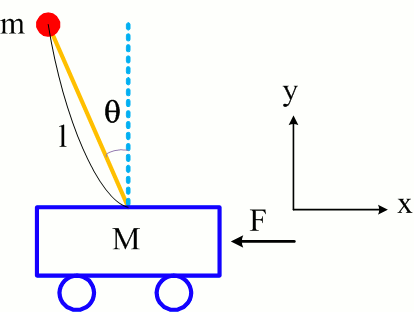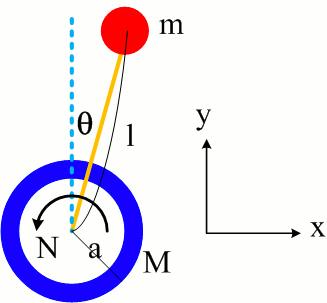Unicycle and Inverted Pendulum

Jongmin Lee December 14, 2007

(Submitted as coursework for Physics 210, Stanford University, Autumn 2007)Fig. 1: Inverted Pendulum

Introduction

A unicycle is an one-wheeled vehicle controlled by a rider's pedaling or wheel-rotation motors [1-4]. You might see a unicycle at least one time in a circus show, and the stabilization of a unicycle seems to be more difficult than that of a normal bike. The principle of a unicycle is similar to that of an inverted pendulum [5,6]. The rider's pedalling in a unicycle problem, a wheel torque, stabilizes the unicycle in the plane of the wheel. The movement of a mobile platform in an inverted pendulum problem, a linear translational force, affects the stabilization of the pendulum. In this article, we will discuss the simplified models of the inverted pendulum and the unicycle.

Inverted Pendulum Equation of Motion

When we think about an inverted pendulum  on a moving platform, we describe it using the following Lagrangian. We define the vertical direction as the y-axis and the horizontal direction as the x-axis. x(t) is the horizontal displacement of a moving platform. The angle from the vertical y-axis is defined as θ(t), which is a function of time. The length of a pendulum is l. The kinetic energy of the system is determined by the kinetic energy of a pendulum 1/2 mv22 and the kinetic energy of a mobile platform 1/2 Mv12. The potential energy of a pendulum is m g l cosθ. The Lagrangian of a simplified inverted pendulum is

L = T - U = 1/2 Mv12 + 1/2 mv22 - m g l cos θ

v1 has only x component, and v2 has x and y component. v2 is explained by x and θ. Substituting

v12 = x'2
v22 = [d/dt (x + l sin θ)]2 + [d/dt (l cos θ)]2 = x'2+2 x' l θ' cos θ + l2θ'2

we obtain

L = 1/2 (M + m)x'2 + m l cos θ x' θ' + 1/2 m l2 θ'2 - m g l cos θ

From the above Lagrangian relations, we can derive the following equations. A linear translational force F causes the feedback motion of a mobile platform, and it stabilizes the motion of an inverted pendulum.

d/dt (∂L/∂x') - ∂L/∂x = (M + m) x'' + m l θ'' cos θ - m l θ'2 sin θ = F
d/dt (∂L/∂θ') - ∂L/∂θ = m l (-g sin θ + x''cos θ + l θ'') = N = 0

Therefore, we get an simplified inverted pendulum's equation of motion.

l [1 - (m / (M + m)) cos2 θ] θ'' + l (m / (M + m)) sin θ cos θ θ'2 - g sin θ = - F / (M + m)

If we know θ'', θ', and θ with motion sensors, the feedback force, F (θ'', θ', θ), enables the inverted pendulum to be the equilibrium state.

When F (θ'', θ', θ) = (1 + ε) m l cos θ2 θ'' - m l sin θ cos θ θ'2, the inverted pendulum's equation of motion becomes a simple form as follows.

l [1 + ε (m / (M + m)) cos2 θ] θ'' - g sin θ = 0Fig. 2: Unicycle

Here, near the equilibrium state, θ ~ 0, cos &theta ~ 1, sin &theta ~ θ and F (θ'', θ', θ) ~ A θ'' - B θ θ' 2 where A and B are constants.

Roughly speaking, as the angle θ' and θ becomes larger, the amplitude of feedback force F has to be larger for stabilizing the inverted pendulum.

Unicycle Equation of Motion

We suppose that a unicycle is composed of a body with mass m, a frame with a massless rod l, and a wheel with mass M and radius a. We neglects roll, yaw, and friction, and we only considered the motion in the plane of wheel. The kinetic energies of a wheel have the components of the translational energy, 1/2 Mv12 and the rotation energy, 1/2 Iω12. The body has the kinetic energy, 1/2 mv22, and the potential energy, m g l cos θ. The Lagrangian of a simplified unicycle is

L = T - U = 1/2 Mv12 + 1/2 Iω12 + 1/2 mv22 - m g l cos θ

where the inertia momenta of a wheel is I = M a2. Substituting

v1 = x' = a ω1 = a θ'
v22 = [d/dt (x + l sin &theta)]2 + [d/dt (l cos θ)]2

we obtain

L = 1/2 (2M + m) x'2 + m l x' θ' cos θ + 1/2 m l2θ'2 - m g l cos θ

From the above Lagrangian relations, we can derive the following equations. A torque N causes the feedback motion of a wheel, and it stabilizes the motion of a unicycle.

d/dt (∂L/∂x') - ∂L/∂ x = (2M + m) x'' + m l ∂2/∂t2 (sin θ) = F = 0
d/dt (∂L/∂θ') - ∂L/∂θ = m l (- g sinθ + x''cos θ + l θ'') = N

Therefore, we get a simplified unicycle equation of motion

[m l2 - (m2 l2 / (2M + m)) cos2 θ] θ'' - (m2 l2 / (2M + m)) sin θ cos θ θ'2 - m g l sin θ = N

If we know θ'', θ', and θ with motion sensors, the feedback torque, N (θ'', θ', θ), could reach the equilibrium state of the unicycle motion. When N (θ'', θ', θ) = - (1 + ε)(m2 l2 / (2M + m)) cos θ2) θ'' - (m2 l2 / (2M + m)) sin θ cos θ θ'2, the unicycle equation of motion reduces to

(l + ε (m l / (2M + m)) cos θ2) θ'' - g sin θ = 0

Here, near the equilibrium state, θ ~ 0, cos &theta ~ 1, sin &theta ~ θ and N ~ - A θ'' - B θ θ'2 where A and B are constants.

Roughly speaking, as the angle θ' and θ becomes larger, the amplitude of feedback torque N has to be larger for stabilizing the unicycle.

Conclusion

We have analyzed the simple models of an inverted pendulum and a unicycle. For stabilizing the unicycle (or the inverted pendulum), we need some feedback such as a wheel torque (or a linear translational force). The linear force F (θ'', θ', θ) comes from the linear movement of a mobile platform by external forces or electric motors. The wheel torque N (θ'', θ', θ) is caused by the rider's pedalling or the wheel-rotation motors.

© 2007 Jongmin Lee. The author grants permission to copy, distribute and display this work in unaltered form, with attribution to the author, for noncommercial purposes only. All other rights, including commercial rights, are reserved to the author.

References

 R. Nakajima, T. Tsubouchi, S. Yuta, E. Koyanagi, "A Development of a New Mechanism of an Autonomous Unicycle", IEEE Proc. on Intelligent Robots and Systems, Grenoble (IEEE, 1997), p. 906

 D. W. Vos, A. H. von Flotow, "Dynamics and Nonlinear Adaptive Control of an Autonomous Unicycle: Theory and Experiment", IEEE Proc. on Decision and Control, Tuscon (1990), p. 182.

 M. Aicardi, G. Casalino, A. Bicchi, A. Balestrino, "Closed Loop Steering of Unicycle-like Vehicles via Lyapunov Techniques", IEEE Robotics & Automation Magazine, 2, 27 (1995).

 R. C. Johnson, "Unicycles and Bifurcations," Am. J. Phys. 66, 589 1998.

 Wikipedia, "Inverted pendulum", http://en.wikipedia.org/wiki/Inverted_pendulum

 C. W. Anderson, "Learning to Control an Inverted Pendulum Using Neural Networks", IEEE Control Systems Magazine, 9, 31 (1989).